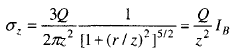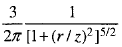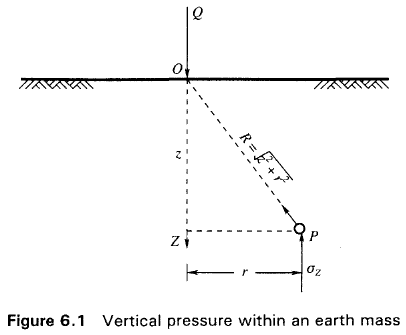Boussinesq’s Theory

# Boussinesq’s Theory - Notes | Study Foundation Engineering - Civil Engineering (CE)

 Table of contentsIntroduction1 Crore+ students have signed up on EduRev. Have you?

Introduction

Estimation of vertical stresses at any point in a soil-mass due to external vertical loadings are of great significance in the prediction of settlements of buildings, bridges, embankments and many other structures. Equations have been developed to compute stresses at any point in a soil mass on the basis of the theory of elasticity. According to elastic theory, constant ratios exist between stresses and strains. For the theory to be applicable, the real requirement is not that the material necessarily be elastic, but there must be constant ratios between stresses and the corresponding strains. Therefore, in non-elastic soil masses, the elastic theory may be assumed to hold so long as the stresses induced in the soil mass are relatively small. Since the stresses in the subsoil of a structure having adequate factor of safety against shear failure are relatively small in comparison with the ultimate strength of the material, the soil may be assumed to behave elastically under such stresses.
When a load is applied to the soil surface, it increases the vertical stresses within the soil mass. The increased stresses are greatest directly under the loaded area, but extend indefinitely in all directions. Many formulas based on the theory of elasticity have been used to compute stresses in soils. They are all similar and differ only in the assumptions made to represent the elastic conditions of the soil mass. The formulas that are most widely used are the Boussinesq and Westergaard formulas. These formulas were first developed for point loads acting at the surface. These formulas have been integrated to give stresses below uniform strip loads and rectangular loads.

The extent of the elastic layer below the surface loadings may be any one of the following:

• Infinite in the vertical and horizontal directions.
• Limited thickness in the vertical direction underlain with a rough rigid base such as a rocky bed.

The loads at the surface may act on flexible or rigid footings. The stress conditions in the elastic layer below vary according to the rigidity of the footings and the thickness of the elastic layer. All the external loads considered in this book are vertical loads only as the vertical loads are of practical importance for computing settlements of foundations.

Figure 6.1 shows a load Q acting at a point 0 on the surface of a semi-infinite solid. A semi-infinite solid is the one bounded on one side by a horizontal surface, here the surface of the earth, and infinite in all the other directions. The problem of determining stresses at any point P at a depth z as a result of a surface point laod was solved by Boussinesq (1885) on the following assumptions.

• The soil mass is elastic, isotropic, homogeneous and semi-infinite.
• The soil is weightless.

The soil is said to be isotropic if there are identical elastic properties throughout the mass and in every direction through any point of it. The soil is said to be homogeneous if there are identical elastic properties at every point of the mass in identical directions.

The expression obtained by Boussinesq for computing vertical stress σz at point P (Fig. 6.1) due to a point load Q is(6.1)
where, r = the horizontal distance between an arbitrary point P below the surface and the vertical axis through the point load Q.
z = the vertical depth of the point P from the surface.
IB ­ Boussines q stres s coefficien t =(6.1a)
The values of the Boussinesq coefficient lB can be determined for a number of values of r/z. The variation of IB with r/z in a graphical form is given in Fig. 6.2. It can be seen from this figurethat IB has a maximum value of 0.48 at r/z = 0, i.e., indicating thereby that the stress is a maximum below the point load.

The document Boussinesq’s Theory - Notes | Study Foundation Engineering - Civil Engineering (CE) is a part of the Civil Engineering (CE) Course Foundation Engineering.
All you need of Civil Engineering (CE) at this link: Civil Engineering (CE)

## Foundation Engineering

18 videos|27 docs|20 tests

## Foundation Engineering

18 videos|27 docs|20 tests

Track your progress, build streaks, highlight & save important lessons and more!

,

,

,

,

,

,

,

,

,

,

,

,

,

,

,

,

,

,

,

,

,

;